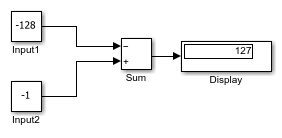# Sum Block Reorders Inputs

This example shows how the Sum block reorders inputs. If you use a `-` sign as the first operation, the block reorders the inputs, if possible, to use a `+` operation. For example, in the expression `output = -a-b+c`, the Sum block reorders the input so that `output = c-a-b`. To initialize the accumulator, the Sum block uses the first `+` input port.

The block avoids performing a unary minus operation on the first operand `a` because doing so can change the value of `a` for fixed-point data types. In that case, the output value differs from the result of accumulating the values for `a` , `b` , and `c` .Both the constant inputs use `int8` data types The Sum block also uses `int8` for the accumulator and output data types and has Saturate on integer overflow turned on. The Sum block reorders the inputs to give the ideal result of `127`.

1. Reorders inputs from `(-Input1 + Input2)` to `(Input2 - Input1)`.

2. Initializes the accumulator by using the first `+` input port. `Accumulator = int8(-1) = -1`

3. Continues to accumulate values. `Accumulator = Accumulator - int8(-128) = 127`

4. Calculates the block output. `Output = int8(127) = 127`

If the Sum block does not reorder the inputs, then you get the nonideal result of `126`.

1. Initializes the accumulator by using the first input port. `Accumulator = int8(-(-128)) = 127`

2. Because saturation is on, the initial value of the accumulator saturates at `127` and does not wrap.

3. Continues to accumulate values. `Accumulator = Accumulator + int8(-1) = 126`

4. Calculates the block output. `Output = int8(126) = 126`

To explicitly specify a unary minus operation for `output = -a-b+c`, you can use the Unary Minus block in the Math Operations library.